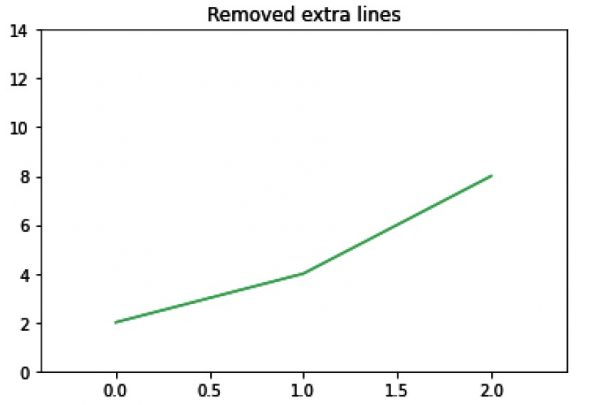# How to remove lines in a Matplotlib plot?

MatplotlibServer Side ProgrammingProgramming

We will create two lines, i.e., line1 and line2. After that, we will pop the second line and will remove it.

## Steps

• Create lists for line1 and line2.

• Plot line1 and line 2 using plot() method, line 2 with style =’dashed’.

• Set or retrieve auto-scaling margins(0.2).

• Pop line 2, and remove it using remove() method.

• The final figure will have only one line, so use plt.show() method.

## Example

import matplotlib.pyplot as plt

line1 = [2, 4, 8]
line2 = [3, 6, 12]

plt.plot(line1)
line_2 = plt.plot(line2, linestyle='dashed')
plt.margins(0.2)
plt.title("With extra lines")
plt.show()

plt.plot(line1)
l = line_2.pop(0)
l.remove()
plt.margins(0.2)
plt.title("Removed extra lines")

plt.show()

## Input Diagram (Before removal of lines)## Output Diagram